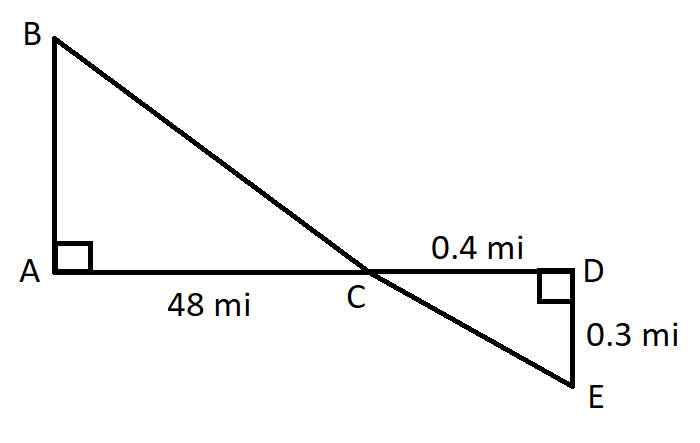# Get help with right triangles and trigonometry

Recent questions in Right triangles and trigonometryKaycee Roche 2021-02-25 Answered

### If a right triangle has one side of 7 and another side of 8, what is the length of the hypotenuse?BenoguigoliB 2021-02-25 Answered

### Can you draw two triangles each having two ${45}^{\circ }$ angles and one ${90}^{\circ }$ angle that are not similar? Justify your answer.ediculeN 2021-02-24 Answered

### Find the length of the leg of the triangle 45-45-90 with a long hypotenuse of 25 inches.Jaden Easton 2021-02-24 Answered

### A wooden block with mass 1.50 kg is placed against a compressed spring at the bottom of an incline of slope 30.0 degrees. When the spring is released, it projects the blockup the incline. At point B, a distance of 6.00 m up the incline from A, the block is moving up te incline at 7.00 m/s andit is no longer in contact with the spring. The coeffiicient of kinetic friction between the block and incline is k =0.50. The mass of the spring is negligible. Calculate the amount of potential energy that wasinitially stored in the spring.Tyra 2021-02-21 Answered

### Use a protractor to measure $\angle a,\angle b$, and $\angle c$. List the angle measures.sjeikdom0 2021-02-14 Answered

### An equilateral triangle has an altitude that measures 6√3 cm. What is the perimeter of this triangle?banganX 2021-02-13 Answered

### Determine whether the set have a subset relationship. Are the two sets disjoint or equivalent? Do the set intersect? R={right triangles}, O={obtuse triangles}permaneceerc 2021-02-12 Answered

### For an acute angle A , can cos A be larger than 1? Explain the answer.illusiia 2021-02-09 Answered

### Use similar triangles to find the distance across the river.e1s2kat26 2021-02-09 Answered

### what is the length of the hypotenuse of a right triangle with legs of length 7 and 8he298c 2021-02-05 Answered

### The base of a triangle is 3 ft more than the height. If the area is 14 ft², find the base and the height.Bevan Mcdonald 2021-02-05 Answered

### Determine whether the set have a subset relationship. Are the two sets disjoint or equivalent? Do the set intersect? L={equilateral triangles}, E={equiangular triangles}Zoe Oneal 2021-02-05 Answered

### Hypotenuse = 5cm, need to work out opposite. (angle opposite the opposite is 34 degrees)aflacatn 2021-02-01 Answered

### One side of an equilateral triangle is given. Solve for the other sides. 3 ft 1 in. 93 m 8.4 yd $8\frac{3}{4}cm$slaggingV 2021-01-31 Answered

### What is the perimeter of the triangle below, to the nearest tenth of an inch, if each square on the grid measures 8 inches on each side?zi2lalZ 2021-01-31 Answered

### The triominoes shown are pieces from a game. Classify the shape of a triomino by its sides and angles. What is the measure of the lagest angle? How many sides are congruent?ediculeN 2021-01-31 Answered

### Two right triangles are similar. The legs of the smaller triangle have lengths of 3 and 4. The scale factor is 1:3. Find the length of the hypotenuse of the larger triangle.Tammy Todd 2021-01-31 Answered

### If Find $\mathrm{sin}\theta$Nannie Mack 2021-01-27 Answered

### If $8{R}^{2}={a}^{2}+{b}^{2}+{c}^{2}$ ,prove that the triangle is right angle triangle. Here a,b and c are the lengths of side of triangle and R is circum radius.arenceabigns 2021-01-25 Answered